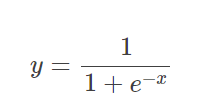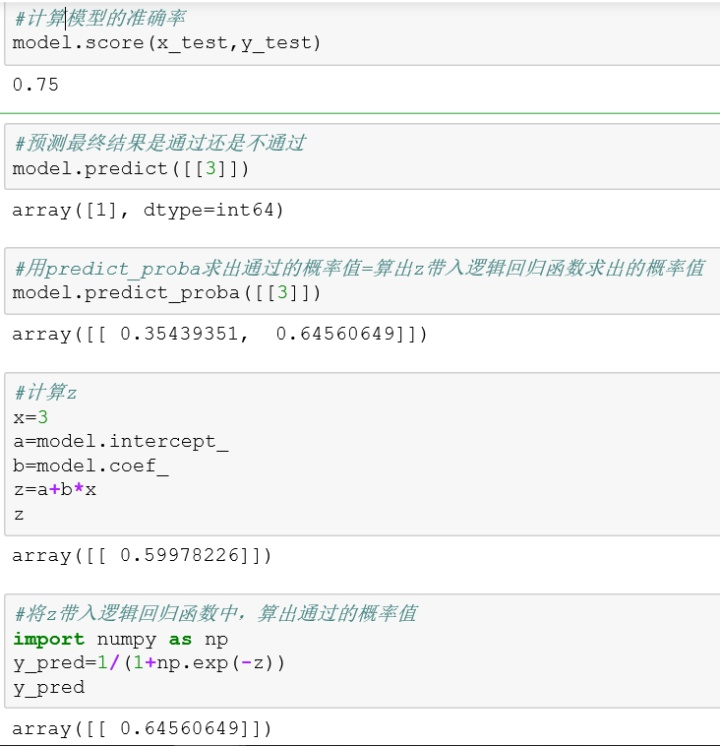python 逻辑回归_Python逻辑回归——建模-评估模型

## 逻辑回归建模-评估模型的过程

1.字典是无序的，所以引入一个OrderedDict来让顺序变成有序

2.数据集转成Pandas的二维数据结构进行处理

## 如何实现逻辑回归

### 2.建立训练数据和测试数据

train_test_split三个参数：

x_train , x_test , y_train , y_test 各分配到一定比例的数据

### 3.使用训练数据训练模型

model.fit(x_train , y_train)

### 4.使用测试数据进行模型评估

model.score(x_test,y_test)

### 5.进一步理解什么是逻辑函数

1）用predict_probe获取概率值

model.predict_proba([])

2）用逻辑回归函数获取概率值z=a+bx

a:截距 a=model.intercept_

b:回归系数 b=model.coef_

y_pred=1/(1+np.exp(-z))

e:通过Numpy中的exp函数求得`#建立有序的二维数据结构import pandas as pdfrom collections import OrderedDictexamDict={    '学习时间':[0.50,0.75,1.00,1.25,1.50,1.75,1.75,2.00,2.25,2.50,            2.75,3.00,3.25,3.50,4.00,4.25,4.50,4.75,5.00,5.50],    '通过考试':[0,0,0,0,0,0,1,0,1,0,1,0,1,0,1,1,1,1,1,1]}examOrder=OrderedDict(examDict)examDf=pd.DataFrame(examOrder)examDf.head() #1.提取出特征和标签x_exam=examDf.loc[:,'学习时间']y_exam=examDf.loc[:,'通过考试']  #2.建立训练数据和测试数据from sklearn.model_selection import train_test_splitx_train, x_test, y_train, y_test=train_test_split(x_exam, y_exam, train_size=0.8)print(x_exam.shape)print(y_exam.shape)print(x_train.shape)print(x_test.shape)print(y_train.shape)print(y_test.shape)  #绘制测试数据和训练数据的散点图import matplotlib.pyplot as pltplt.scatter(x_train, y_train, color="blue", label="train data")plt.scatter(x_test, y_test, color="red", label="test data")plt.legend(loc=2)plt.xlabel("Hours")plt.ylabel("Pass")plt.show()  #3.使用训练数据训练模型x_train=x_train.reshape(-1,1)y_train=y_train.reshape(-1,1) from sklearn.linear_model import LogisticRegressionmodel=LogisticRegression()model.fit(x_train, y_train) #4.使用测试数据进行模型评估x_test=x_test.reshape(-1,1)y_test=y_test.reshape(-1,1)model.score(x_test,y_test)  #5.计算模型的准确率model.score(x_test,y_test) #预测最终结果是通过还是不通过model.predict([]) #用predict_proba求出通过的概率值=算出z带入逻辑回归函数求出的概率值#计算概率值得方法1model.predict_proba([])#计算概率值得方法2x=3a=model.intercept_b=model.coef_z=a+b*x#将z带入逻辑回归函数中，算出通过的概率值import numpy as npy_pred=1/(1+np.exp(-z))y_pred`

#### 最新留言

[***.144.177.141]2023年09月15日 15时05分39秒